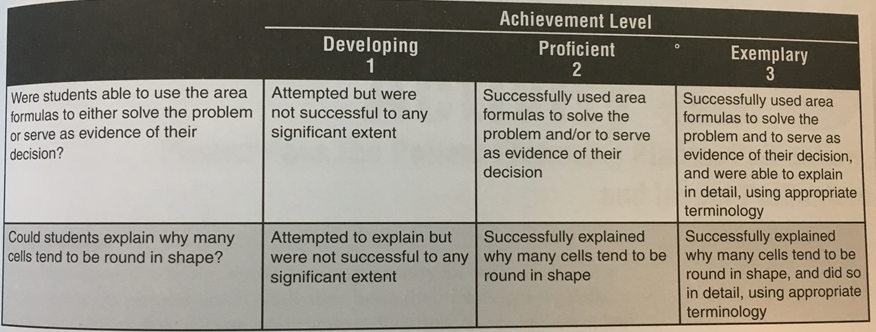Exploring Cellular Shape Using Area
starstarstarstarstarstarstarstarstarstar
by Katie Pierson
| 10 Questions
Note from the author:
Students use area formulas to prove why most cells are round or oval.
Learning Target: I can use area formulas of circle, square, rectangle, and triangle to explore cellular shape.

1
1
Draw a picture of a cell and describe its shape.
All living things are made of cells (this concept is known as cell theory). Cells usually need maximum surface area (The total area of the surface of a three-dimensional object. Example: the surface area of a cube is the area of all 6 faces added together) for transfer and exchange of materials across the cell membrane. Given a certain amount of surface area for a particular, single cell, what general shape must the cell be to maximize its volume? The answer in this question lies in the cell's general shape. To answer this question, we will be working in two dimentsions rather than three for the sake of simplicity.
Challenge: Each group must make as large a cell as possible with the string, using any of the four basic geometric shapes (circle, square, rectangle, or triangle). You must be able to prove that your shape solution is largest using the formulas for area below.

Materials: 1m of string, 1 meter stick, calculators2
1
What is the area of your cell that is in the shape of a circle?
3
1
What is the area of your cell that is in the shape of a rectangle?
4
1
What is the area of your cell that is in the shape of a square?
5
1
What is the area of your cell that is in the shape of a triangle?
Discussion Questions
6
1
Would you be surprised to find that many cells are round or oval shaped? Explain your answer.
7
1
How are area formulas useful? Can you think of some ways that they might be useful to you or to people you know?
8
1
How do real cells differ from the one you "made" with your string membrane?Extension
9
1
Not all cells are round or oval. Can you discover some cells that have other shapes? Are their shapes related to their functions, and if so, how? (Consider epithelial cells, neurons, or red blood cells)
10
1
If you wanted to make a chicken coop and you had only 10 yd. of chicken wire to use, what shape would make the coop as big as possible? Explain.